Tamilnadu State Board New Syllabus Samacheer Kalvi 11th Maths Guide Pdf Chapter 3 Trigonometry Ex 3.8 Text Book Back Questions and Answers, Notes.

## Tamilnadu Samacheer Kalvi 11th Maths Solutions Chapter 3 Trigonometry Ex 3.8

Question 1.
Find the principal solution and general solutions of the following
(i) sin θ = – $$\frac{1}{\sqrt{2}}$$
(ii) cot θ = √3
(iii) tan θ = –$$\frac{1}{\sqrt{3}}$$
(i) sin θ = – $$\frac{1}{\sqrt{2}}$$
We know that principal of sin θ lies in $$\left[-\frac{\pi}{2}, \frac{\pi}{2}\right]$$
sin θ = – $$\frac{1}{\sqrt{2}}$$ < 0
∴ The principal value of sin θ lies in the IV quadrant.
sin θ = – $$\frac{1}{\sqrt{2}}$$
= – sin $$\left(\frac{\pi}{4}\right)$$
sin o = sin $$\left(-\frac{\pi}{4}\right)$$
Hence θ = $$-\frac{\pi}{4}$$ is the principal solution.
The general solution is
θ = nπ + (- 1)n . $$\left(-\frac{\pi}{4}\right)$$ , n ∈ Z
θ = nπ + (- 1)n + 1 . $$\frac{\pi}{4}$$ , n ∈ Z(ii) cot θ = √3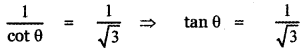The principal value of tan θ lies in $$\left(-\frac{\pi}{2}, \frac{\pi}{2}\right)$$
Since tan θ = $$\frac{1}{\sqrt{3}}$$ > 0
The principal value of tan θ lies in the I quadrant.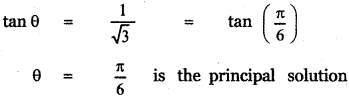The general solution of tan θ is
θ = nπ + $$\frac{\pi}{6}$$ , n ∈ Z

(iii) tan θ = –$$\frac{1}{\sqrt{3}}$$
The principal value of tan θ lies in $$\left(-\frac{\pi}{2}, \frac{\pi}{2}\right)$$
Since tan θ = – $$\frac{1}{\sqrt{3}}$$ > 0
The principal value of tan θ lies in the IV quadrant.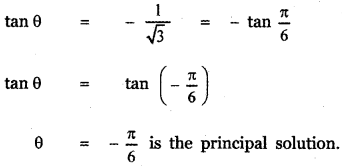The general solution of tan θ is
θ = nπ – $$\frac{\pi}{6}$$ , n ∈ ZQuestion 2.
Solve the following equations for which solutions lies in the interval 0° ≤ 9 < 360°
(i) sin4x = sin2x
sin4x – sin2x = 0
sin2 x (sin2 x – 1) = 0
sin2 x [ – (1 – sin2 x)] = 0
sin2x × – cos2x = 0
– sin2x cos2x = 0
(sin x cos x)2 = 0
($$\frac { 1 }{ 2 }$$ × 2 sin cos x)2 = 0
$$\frac { 1 }{ 4 }$$ sin 2x = 0
sin 2x = 0
The general solution is
2x = nπ, n ∈ Z
x = $$\frac{\mathrm{n} \pi}{2}$$, n ∈ Z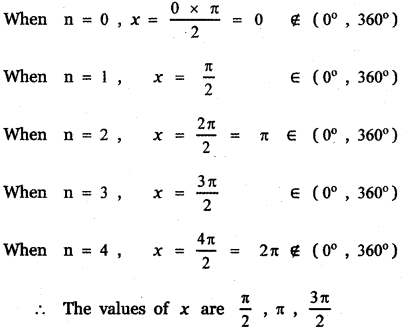(ii) 2 cos2x + 1 = – 3 cos x
2 cos2x + 1 = – 3 cos x
2 cos2x + 3 cos x + 1 = 0
2 cos2x + 2 cos x + cos x + 1 = 0
2 cos x (cos x + 1) + 1 (cos x + 1) = 0
(2 cos x + 1) (cos x + 1) = 0
2 cos x + 1 = 0 or cos x + 1 = 0
cos x = $$-\frac{1}{2}$$ or cos x = – 1
To find the solution of cos x = $$-\frac{1}{2}$$
cos x = $$-\frac{1}{2}$$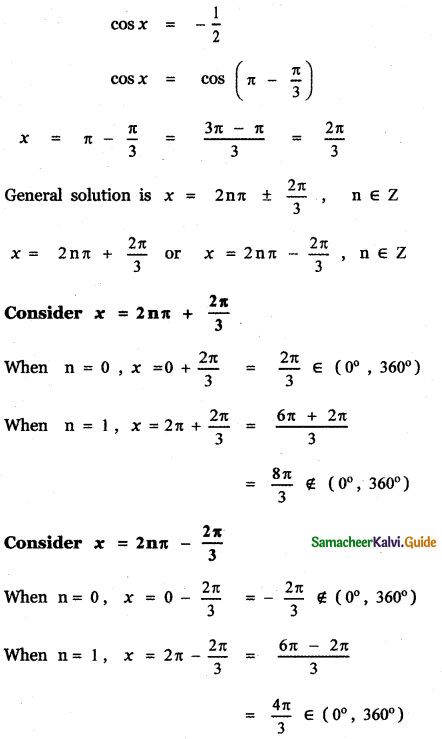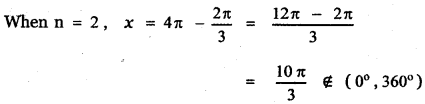To find the solution of cos x = – 1
cos x = – 1
cos x = cos π
The general solution is
x = 2nπ ± π, n ∈ Z
x = 2nπ + π or x = 2nπ – π, n ∈ Z

Consider x = 2nπ + π
when n = 0 , x = 0 + π = π ∈ (0°, 360°)
when n = 1 , x = 2π + π = 3π ∉ (0°, 360°)

Consider x = 2nπ – π
when n = 0, x = 0 – π ∉ (0°, 360°)
when n = 1, x = 2π – π = π ∈ (0°, 360°)
when n = 2, x = 4π – π = 3π ∉ (0°, 360°)
∴ The required solution are x = $$\frac{2 \pi}{3}$$, $$\frac{4 \pi}{3}$$, π(iii) 2 sin2x + 1 = 3 sin x
2 sin2x – 3 sin x + 1 = 0
2 sin2x – 2 sin x – sin x + 1 = 0
2 sin x (sin x – 1) – 1 (sin x – 1) = 0
(2 sin x – 1)(sin x – 1) = 0
2 sin x – 1 = 0 or sin x – 1 = 0
sin x = $$\frac { 1 }{ 2 }$$ or sin x = 1
To find the solution of sin x = $$\frac { 1 }{ 2 }$$
sin x = $$\frac { 1 }{ 2 }$$
sin x = sin $$\left(\frac{\pi}{6}\right)$$
The general solution is x = nπ + (-1)n$$\frac{\pi}{6}$$, n ∈ z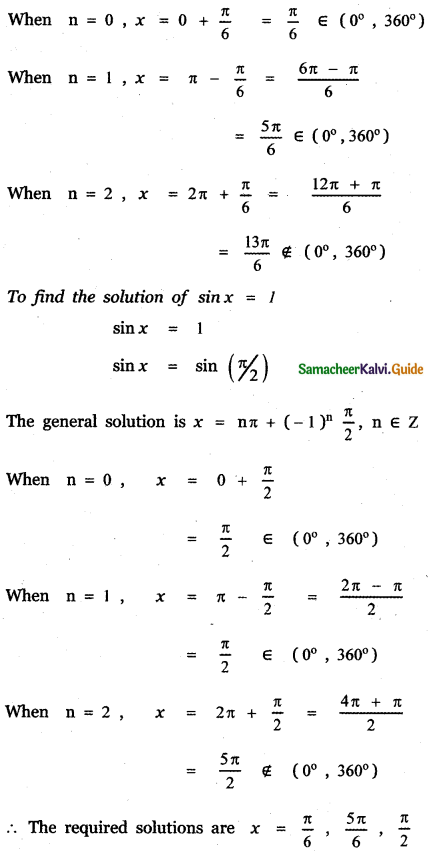(iv) cos2x = 1 – 3 sin x
1 – 2 sin2x = 1 – 3 sinx
2 sin2 x – 3 sin x = 0
sin x(2 sin x – 3) = 0smx =
sin x = 0 or 2 sin x – 3 = 0
sin x = 0 or sin x = $$\frac{3}{2}$$
sin x = $$\frac{3}{2}$$ is not possible since sin x ≤ 1
∴ sin x = 0 = sin 0
The general solution is x = nit ,
When n = 0, x = 0 ∉ (0°, 360°)
When n = 1, x = π ∈ (0°, 360°)
When n = 2, x = 2π ∉ (0°, 360°)
∴ The required solutions is x = πQuestion 3.
Solve the following equations:
(i) sin 5x – sin x = cos 3x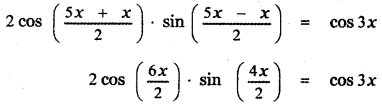2 cos 3 x . sin 2x = cos 3 x
2 cos 3x . sin 2x – cos3x = 0
cos 3x (2 sin 2x – 1) = 0
cos 3x = 0 or 2 sin 2x – 1 = 0
cos 3x = 0 or sin 2x = $$\frac { 1 }{ 2 }$$
To find the general solution of cos 3x = 0
The general solution of cos 3x = 0 is
3x = (2n + 1)$$\frac{\pi}{2}$$, n ∈ Z
x = (2n + 1)$$\frac{\pi}{6}$$, n ∈ Z
To find the general solution of sin 2x = $$\frac{1}{2}$$
sin 2x = $$\frac{1}{2}$$
sin 2x = sin $$\left(\frac{\pi}{6}\right)$$
The general solution is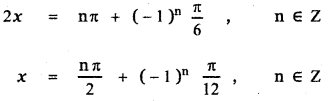∴ The required solutions are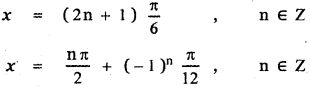(ii) 2 cos2θ + 3 sin θ – 3 = θ
2 cos2θ + 3 sin θ – 3 = θ
2(1 – sin2θ)+ 3 sin θ – 3 = θ
2 – 2 sin2θ + 3 sin θ – 3 = θ
– 2 sin2θ + 3 sin θ – 1 = θ
2 sin2 θ – 3 sin θ + 1 = θ
2 sin2θ – 2 sin θ – sin θ + 1 = θ
2 sin θ (sin θ – 1) – (sin θ – 1) = θ
(2 sin θ – 1) (sin θ – 1) = 0
2 sin θ – 1 = 0 or sin θ – 1 = θ
sin θ = $$\frac { 1 }{ 2 }$$ or sin θ = 1
To find the general solution of’ sin θ = $$\frac { 1 }{ 2 }$$
sin θ = $$\frac { 1 }{ 2 }$$
sin θ = sin $$\frac{\pi}{6}$$
The general solution is θ = nπ + (-1)n$$\frac{\pi}{6}$$, n ∈ Z
To find the general solution of sin θ = 1
sin θ = 1
sin θ = $$\frac{\pi}{2}$$
The general solution is θ = nπ + (-1)n$$\frac{\pi}{2}$$, n ∈ Z
∴ The required solutions are
θ = nπ + (-1)n$$\frac{\pi}{6}$$, n ∈ Z (or)
θ = nπ + (-1)n$$\frac{\pi}{6}$$, n ∈ Z(iii) cos θ + cos 3θ = 2 cos 2θ
cos 3θ + cos θ = 2 cos 2θ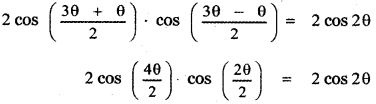2 cos 2θ . cos θ = 2 cos 2θ
cos 2θ . cos θ – cos 2θ = θ
cos 2θ (cos θ – 1) = θ
cos 2θ = θ or cos θ – 1 = θ
cos 2θ = θ or cos θ = 1
To find the general solution of cos 2θ = θ
The general solution is
2θ = (2n + 1)$$\frac{\pi}{2}$$, n ∈ Z
θ = (2n + 1)$$\frac{\pi}{4}$$, n ∈ Z
To find the general solution of cos θ = 1
cos θ = 1
cos θ = cos 0
The general solution is θ = 2nπ , n ∈ Z
∴ The required solutions are
θ = (2n + 1)$$\frac{\pi}{4}$$, n ∈ Z (or)
θ = 2nπ, n ∈ Z(iv) sin θ + sin 3θ + sin 5θ = 0
sin 5θ + sin 3θ + sin θ = 0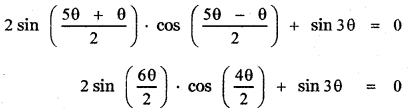2 sin 3θ . cos 2θ + sin 3θ = 0
sin 3θ (2 cos 2θ + 1) = θ
sin 3θ = 0 or 2 cos 2θ + 1 = θ
sin 3θ = 0 or cos 2θ = –$$\frac { 1 }{ 2 }$$
To find the general solution of sin 3θ = 0
The general solution is
3θ = nπ, n ∈ Z
θ = $$\frac{\mathbf{n} \pi}{3}$$, n ∈ Z
To find the general solution of cos 2θ = –$$\frac { 1 }{ 2 }$$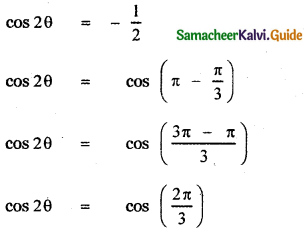The general solution is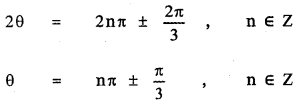∴ The required solutions are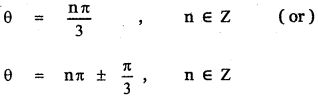(v) sin 2θ – cos 2θ – sin θ + cos θ = θ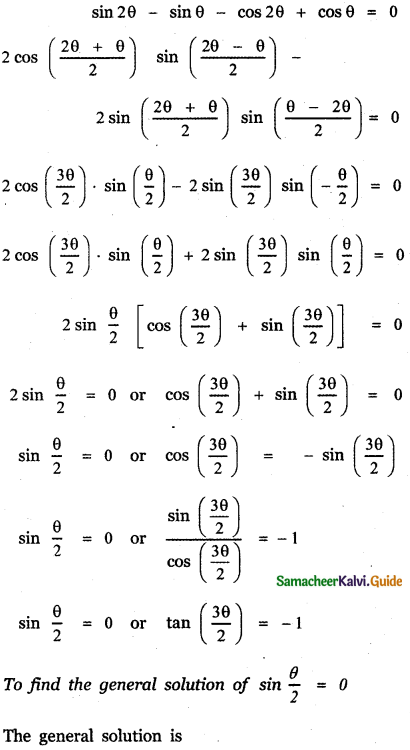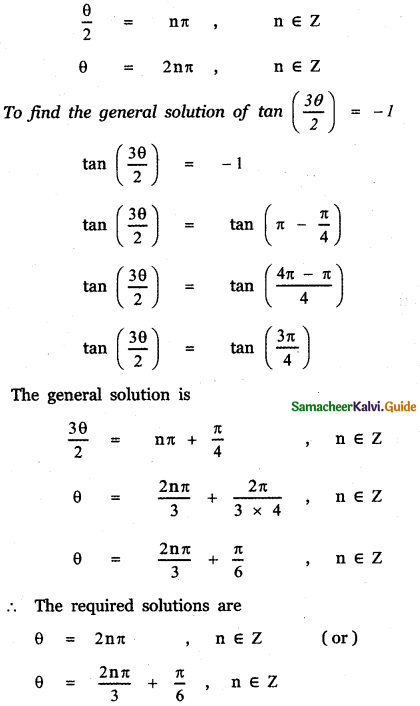(vi) sin θ + cos θ = √2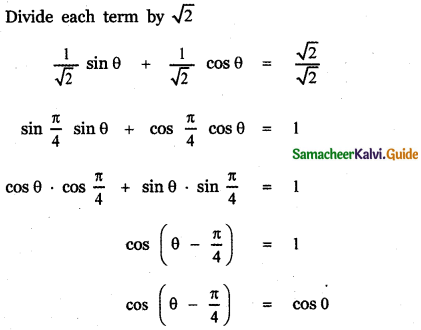The general solution is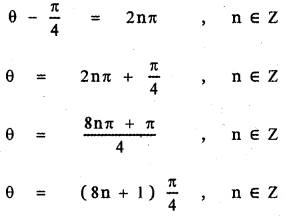(vii) sin θ + √3 cos θ = 1
Divide each term by 2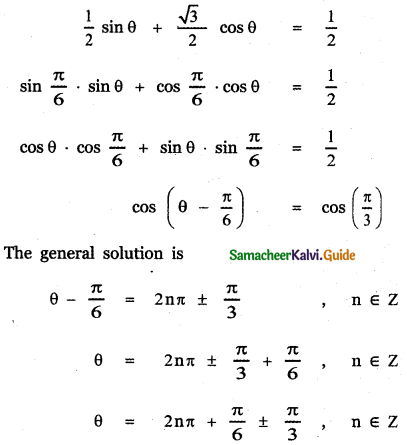(viii) cot θ + cosec θ = √3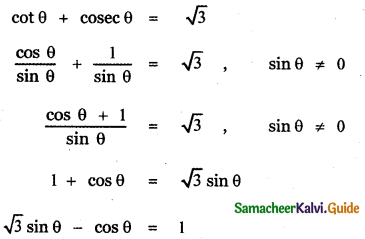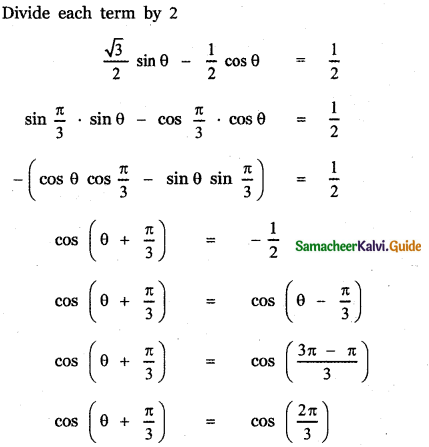(ix) tan θ + tan $$\left(\theta+\frac{\pi}{3}\right)$$ + tan $$\left(\theta+\frac{2 \pi}{3}\right)$$ = √3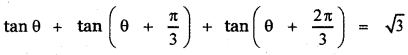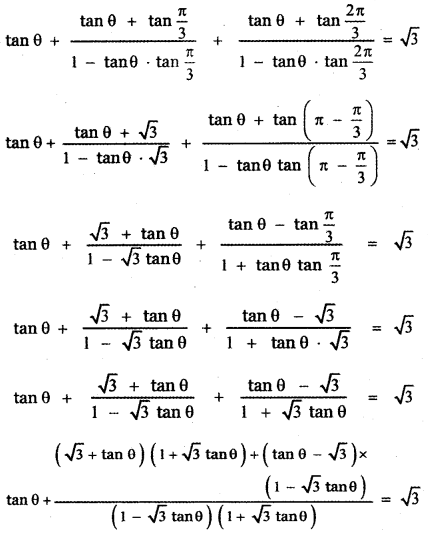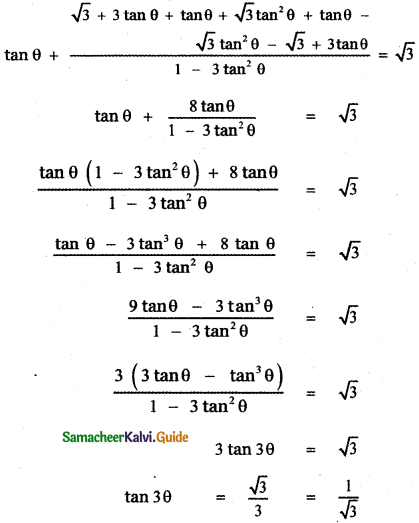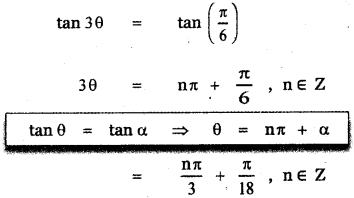(x) cos 2θ = $$\frac{\sqrt{5}+1}{4}$$
we know cos 36° = $$\frac{\sqrt{5}+1}{4}$$, 36° = $$\frac{\pi}{5}$$
cos 2θ = cos 36° = cos $$\left(\frac{\pi}{5}\right)$$
The general solution is
2θ = 2nπ ± $$\frac{\pi}{5}$$, n ∈ Z
θ = nπ ± $$\frac{\pi}{10}$$, n ∈ Z(xi) 2cos 2x – 7 cos x + 3 = 0
cos x = $$\frac { 1 }{ 2 }$$ or cos x = 3
∴ cos x = $$\frac { 1 }{ 2 }$$
cos x = cos $$\frac{\pi}{3}$$
The general solution is x = 2nπ ± $$\frac{\pi}{3}$$, n ∈ Z